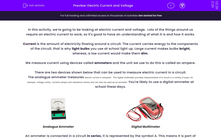# Compare Current and Voltage

In this worksheet, students will learn what electric current and voltage are and how we measure them.Key stage:  KS 3

Curriculum topic:   Physics: Electricity and Electromagnetism

Curriculum subtopic:   Current Electricity

Popular topics:   Electricity worksheets

Difficulty level:#### Worksheet Overview

In this activity, we're going to be looking at electric current and voltage.  Lots of the things around us require an electric current to work, so it's good to have an understanding of what it is and how it works.

Current is the amount of electricity flowing around a circuit. The current carries energy to the components of the circuit; that is why light bulbs you use at school light up. Large current makes bulbs bright, whereas a low current would make them dim.

We measure current using devices called ammeters and the unit we use to do this is called an ampere.

There are two devices shown below that can be used to measure electric current in a circuit.

The analogue ammeter measures electric current in amperes. The digital multimeter provides measurements of a circuit in a variety of ways, for example, voltage (volts), currents (amps) and resistance (ohms) and can also be used as an ammeter. You're likely to use a digital ammeter at school these days.Analogue Ammeter Digital Multimeter

An ammeter is connected in a circuit in series, it is represented by the symbol A. This means it is part of the circuit loop (see diagram). The position of the ammeter does not really matter because the current is the same anywhere in the circuit. Current is not used up as it goes around the circuit. The unit of measuring current is called ampére. We abbreviate this as amps.Current in a circuit can be changed by changing the circuit components. For example, if a circuit has one bulb and the current is 0.3 A, it will drop if we add another bulb. Light bulbs have very small wires in them called filaments (see the orange wire in bulb picture).Filaments make it very hard for the current to flow, so adding another one makes it even harder. We say that filaments carry resistance, which makes it harder for current to go through them. Resistance is measured in Ohms (Ω). The formula used to calculate resistance is:

resistance = voltage / current

Voltage is the measurement of how much energy is transferred by electricity. It is measured in volts (V) with a device called a voltmeter. A voltmeter looks like an ammeter but it measures voltage. Voltmeters are always connected in a circuit in parallel to a component (see example in the diagram: the voltmeter, shown as V, is connected in parallel to the light bulb).Cells have a voltage marked on them. The bigger the voltage, the higher the current. Chemicals inside cells provide the voltage.

### What is EdPlace?

We're your National Curriculum aligned online education content provider helping each child succeed in English, maths and science from year 1 to GCSE. With an EdPlace account you’ll be able to track and measure progress, helping each child achieve their best. We build confidence and attainment by personalising each child’s learning at a level that suits them.

Get started